Browse by Topic
Related Topics

# Mathematica Q&A: Computing Freight-Container Check Digits

Got questions about Mathematica? The Wolfram Blog has answers! We’ll regularly answer selected questions from users around the web. You can submit your question directly to the Q&A Team using this form.

This week’s question comes from Adri, an engineer:

How can I calculate the check digit in freight container codes like MSKU3881107?

We had to start with some quick research for this question: it turns out that freight (shipping) container identification is covered by the ISO 6346 standard (Wikipedia). Under ISO 6346, each container is labeled with an 11-digit code (four letters + seven numerals) in which the last digit is a “check” digit that is computed from the other 10 digits, according to a fixed rule. For example, in MSKU3881107, the final “7” is the check digit.

The rule specified by ISO 6346 for computing the check digit is designed so most accidental changes or misreadings of a single digit in a code will also change the check digit. This means you can use the check digit to catch most such errors; whenever you see a code, you calculate the check digit yourself and see if it matches up with the one in the code.

The calculation of the check digit can be described in three steps. In the first step, the four letters are replaced with corresponding numbers. The letters A through Z correspond to the numbers 10 through 38, with all multiples of 11 skipped: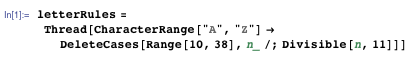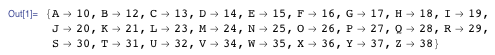Here are the first 10 Characters of the code “MSKU3881107”: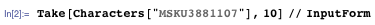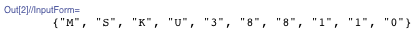Applying the replacement rules above, you get these 10 numbers: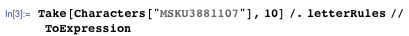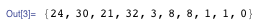(ToExpression converts text characters like “3” into numbers like 3.)

In the second step, the 10 numbers are multiplied by successive powers of two and added together. Here are the successive powers of two: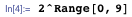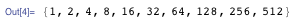The multiplication followed by addition is simply a Dot product: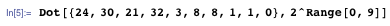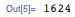In the third step, you take the remainder when dividing by 11, and if the result is 10, it is taken to be zero (though by convention, codes that give either zero or 10 are not normally used). The final result is the check digit: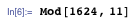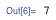As you can see, this check digit agrees with the last digit of the original freight code MSKU3881107.

You might as well wrap this up into a function. Here’s one that supports two different interfaces. The first version accepts a list of numbers like {24, 30, 21, 32, 3, 8, 8, 1, 1, 0} and applies steps 2 and 3: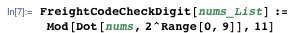The second version accepts a text string like “PONU2079674” and applies step 1, then simply calls the first version to apply steps 2 and 3: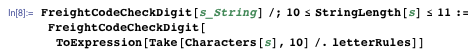Note that, by means of a Condition (/;), the string s is allowed to have either 10 digits (for a code without a check digit) or 11 digits (for a code that already includes a check digit). The result is the same either way: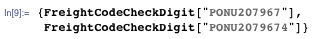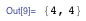Here’s a related function that checks whether a freight code has the correct check digit: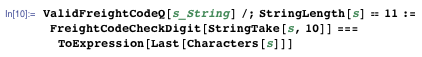Test a few freight codes: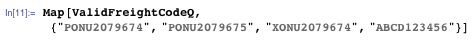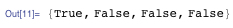This answers the original question. It’s interesting to investigate how well the check digit works at detecting small errors in container codes.

Suppose you change the nth digit in a container code from a to a different value b. The contribution (modulo 11) of the nth digit to the value of the check digit changes from Mod[a 2^n, 11] to Mod[b 2^n, 11]. The contribution of all the other digits stays the same.

You can use the function FindInstance to check whether there are any possible values of a, b, and n such that Mod[a 2^n, 11] == Mod[b2^n, 11]. For numerical digits you can assume 0 ≤ a ≤ 9, 0 ≤ b ≤ 9, and 4 ≤ n ≤ 9 , and you get: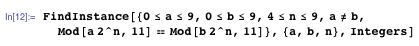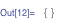This means that a change to any single numerical digit is guaranteed to change the check digit.

What about the four letters? Here you can assume 10 ≤ a ≤ 38, Mod[a, 11] ≠ 0, 10 ≤ b ≤ 38, Mod[b, 11] ≠ 0, and 0 ≤ n ≤ 3, and you get: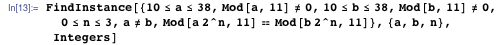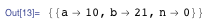This means that the check digit can sometimes fail to detect a change to one of the four letters in the container code. For example, these two codes differ by one letter despite having the same check digit: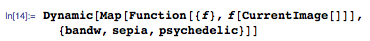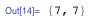The only changes to one of the four letters that will “fool” the check digit are those that, according to letterRules above, differ exactly by a multiple of 11, such as “A” → 10 and “K” → 21. This means most accidental changes to a single letter will still be caught.

Another possible accidental change would be swapping exactly two of the numeric digits in a code—something like “ABCD123456” → “ABCD623451”. Can you work out whether a change like this can ever fool the check digit? What about if codes with check digits of 0 (or 10) are allowed?

If you have a question you’d like answered in this blog, you can submit it to the Q&A Team using this form. For daily bite-sized Mathematica tips, follow our @MathematicaTip Twitter feed (or follow using RSS).